# GMAT Math : Calculating the length of an edge of a cube

## Example Questions

### Example Question #801 : Gmat Quantitative Reasoning

If the volume of a cube isunits cubed, what is the length of each side of the cube?Explanation:

We solve for the side of the cube by deriving it from the volume of a cube formula: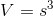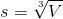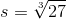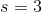### Example Question #802 : Gmat Quantitative Reasoning

If a cube has a surface area of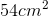, what is the length of one side?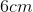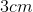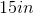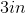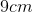Explanation:

To find the surface area of a cube, use the following formula: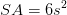Where s is our side length.

Rearrange for s to get the following: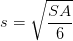Plug in 54 for SA and solve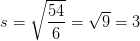Don't forget your units, in this case centimeters so we get 3 centimeters

### Example Question #803 : Gmat Quantitative Reasoning

If a cube has a surface area of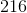, what is the length of one of its sides?Explanation:

To solve for the side length of the cube, we need to use the formula for surface area. There are six faces on a cube, so its total surface area is just six times the area of one of its square faces, which is given by the side length squared: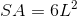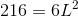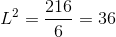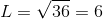### Example Question #804 : Gmat Quantitative Reasoning

If a cube has a volume of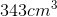and is made up ofsmaller cubes, what is the length of one side of one of the smaller cubes?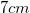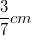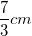Explanation:

Volume of a cube is equal to the cube of its side. 343 is equal to 7 cubed, so the length of the whole cube is 7 cm. The cube is made up of 27 smaller cubes though. That means that one face of the cube is made up of 9 cubes and one edge of the whole cube is made up of 3 small cubes. That means that the length of one small cube is equal to the total length of one side divided by the numbers of cubes per side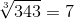Then we get our final answer by doing:### Example Question #805 : Gmat Quantitative Reasoning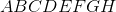is a cube with diagonal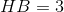. What is the length of an edge of the cube?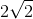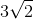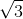Explanation:

We are given the length of the diagonal of the cube.

Therefore we can find the length of an edge by using the formula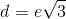or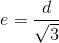, and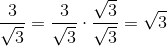.

Therefore, the final answer is.

### Example Question #806 : Gmat Quantitative Reasoning

Find the length of the edge of a cube given that the volume is.Explanation:

To find side length, you must use the equation for volume of a cube and solve for.Thus,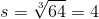Tired of practice problems?

Try live online GMAT prep today.